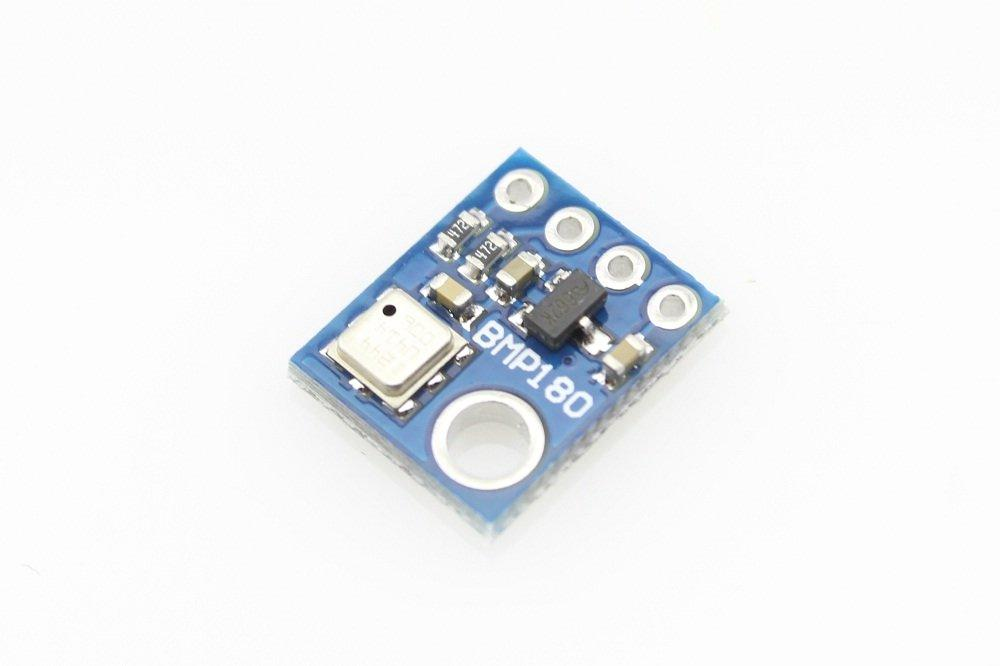# Arduino Due and BMP180 sensor example

86

This bmp180 from Bosch is the best low-cost sensing solution for measuring barometric pressure and temperature. The sensor is soldered onto a PCB with a 3.3V regulator, I2C level shifter and pull-up resistors on the I2C pins. The BMP180 replaces the BMP085.

## Specification

• Pressure sensing range: 300-1100 hPa (9000m to -500m above sea level)
• Up to 0.03hPa / 0.25m resolution
• -40 to +85°C operational range, +-2°C temperature accuracy

Here is a breakout which makes it easy to use the sensor. The sensor will cost less than \$2.## Code

You will need the Adafruit BMP085 library for this example, you can either download it or use the library manager in newer Arduino IDEs.

In this example I am only looking at the temperature and pressure but there are other functions in the library

 Source code```#include <Wire.h>

void setup()
{
Serial.begin(9600);
//Wire.begin (4, 5);
if (!bmp.begin())
{
Serial.println("Could not find BMP180 or BMP085 sensor at 0x77");
while (1) {}
}
}

void loop()
{
Serial.print("Temperature = ");
Serial.println(" Celsius");

Serial.print("Pressure = ");
Serial.println(" Pascal");

Serial.println();
delay(5000);
}```

## Output

Open the Serial monitor and you should see something like this

Temperature = 19.70 Celsius
Pressure = 97855 Pascal

Temperature = 26.40 Celsius
Pressure = 97982 Pascal

Temperature = 29.20 Celsius
Pressure = 98099 Pascal

Temperature = 27.50 Celsius
Pressure = 97864 Pascal

Temperature = 26.10 Celsius
Pressure = 97865 Pascal

Temperature = 25.00 Celsius
Pressure = 97864 Pascal